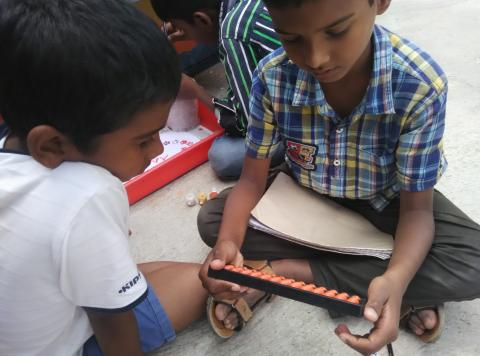# Study Smart - Maths

## Primary tabsSome of us like maths, some are horrified...we explored with Sangeetha how can we study smarter when it comes to maths.

Many times it's not about the operations of maths or to know the calculations but about understanding the problem.
So here are the few steps to approach any maths problem
First Step - Check the units ( numbers or rupees or lengthy or area).
Second Step - Find out what all is given (length, numbers, cost etc)
Third step - What is the problem asking? (Find area or cost or total numbers)
Step Fourth - Draw the diagram if possible.
Step Five - Estimate
Step Six - Solve the problem
Step Seven - Verify or Check ( if possible do it in reverse order).

The steps may allow us to maths with a perspective that 'let's understand', rather than starting with 'I don't know'.